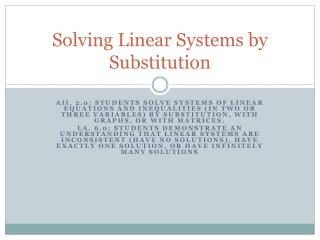DownloadDownload PresentationSolving Linear Systems by Substitution

# Solving Linear Systems by Substitution

Download Presentation## Solving Linear Systems by Substitution

- - - - - - - - - - - - - - - - - - - - - - - - - - - E N D - - - - - - - - - - - - - - - - - - - - - - - - - - -
##### Presentation Transcript

1. Solving Linear Systems by Substitution AII, 2.0: Students solve systems of linear equations and inequalities (in two or three variables) by substitution, with graphs, or with matrices. LA, 6.0: Students demonstrate an understanding that linear systems are inconsistent (have no solutions), have exactly one solution, or have infinitely many solutions

2. Solving Linear Systems by Substitution Objectives Key Words Solve a system of linear equations in two variables by substitution Substitution Method

3. Prerequisite Check:If you do not know, you need to let me know Solve the equation for the indicated variable Evaluate For For For For

4. Steps: • Solve one equation for one of its variables • Substitute the expression from Step 1 into the other equation and solve for the other variable • Substitute the value from Step 2 into the revised equation from Step 1 and solve • Check the solution in each of the original equations Solving a Linear System by Substitution Step-by-Step

5. 4x y 6 = y 2x = SOLUTION Substitute 2x for y in Equation 2. Solve for x. – Write Equation 2. 4x y 6 = – Substitute 2x for y. 4x 2x 6 = 2x 6 Combine like terms. = Solve for x. x 3 = Example 1 Use Substitution Solve the system using substitution. Equation 1 Equation 2

6. Write Equation 1. Substitute 3 for x. ( ) 3, 6 Solve for y. y 2x = y 2 = y 6 = ANSWER The solution is . ( ) 3 Example 1 Use Substitution Substitute 3 for x in Equation 1. Solve for y. You can check your answer by substituting 3 for x and 6 for y in both equations.

7. SOLUTION STEP 1 Solve Equation 2 for x. Choose Equation 2 because the coefficient of x is 1. – – x 2y 3 = – x 2y 3 Solve for x to get revised Equation 2. = Example 2 Use Substitution Solve the system using substitution. Equation 1 + 3x 2y 7 = – – Equation 2 x 2y 3 =

8. Write Equation 1. + 3x 2y 7 = Substitute 2y3 for x. – + 3 2y 7 = Use the distributive property. + 6y 9 – 2y 7 = Combine like terms. ( ) 2y 3 – 8y 9 – 7 = Add 9 to each side. 16 8y = Solve for y. y 2 = Example 2 Use Substitution STEP 2 Substitute2y3 for x in Equation 1. Solve for y. –

9. Write revised Equation 2. x 2y 3 = Substitute 2 for y. – x 2 3 = Simplify. x 1 = ( ) 2 STEP 4 Check by substituting 1 for x and 2 for y in the original equations. Equation 1 Equation 2 – – + 3x 2y 7 x 2y 3 = = Write original equations. Example 2 Use Substitution STEP 3 Substitute2 for y in revised Equation 2. Solve for x.

10. 3 + 2 7 1 2 Simplify. – 1 4 3 + 4 7 Solution checks. 7 7 = = ( ) 1, 2 ANSWER The solution is . ( ( ( ) ) ) 1 2 2 ? ? ? ? – – – – 3 3 3 3 = = = = Example 2 Use Substitution Substitute for x and y.

11. Checkpoint ANSWER + 2x y 3 = . Sample answer: The second equation; this equation had 0 on one side and the coefficient of y was 1, so I solved for y to obtain y3x. ( ) – 3 , 9 + 3x y 0 = – = Use Substitution Solve the system using substitution. Tell which equation you chose to solve and use for the substitution. Explain. 1.

12. Checkpoint + 2x 3y 4 = + x 2y 1 = ANSWER . Sample answer: The second equation; the coefficient of x in this equation was 1, so solving for x gave a result that did not involve any fractions. ( ) – 5, 2 Use Substitution Solve the system using substitution. Tell which equation you chose to solve and use for the substitution. Explain. 2.

13. Checkpoint – 3x y 5 = + 4x 2y 10 = ANSWER . Sample answer: The first equation; the coefficient of y in this equation was 1, so solving for y gave a result that did not involve any fractions. ( ) 2, 1 – Use Substitution Solve the system using substitution. Tell which equation you chose to solve and use for the substitution. Explain. 3.

14. Conclusions Summary Assignment • How do you solve a system of linear equations algebraically? • To use the substitution method sole for one variable and then substitute the value found into the other equations and solve for the second variable. Pg135#(12,18,22,30,31) Due by the end of the class.